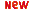The OreMorphisms package is dedicated to the study of homomorphisms of finitely generated left modules over an Ore algebra (available in the Ore_algebra package), and its applications to mathematical systems theory and mathematical physics.

In particular, OreMorphisms package can be used to:

• compute homomorphisms between two finitely generated left modules,
• compute internal symmetries of linear functional systems,
• compute the kernel, coimage, image and cokernel of homomorphisms,
• Fitting's theorem on isomorphisms and equivalences,
• compute idempotent endomorphisms,
• factorize linear functional systems,
• reduction linear functional systems,
• decompose linear functional systems...

For more details, see:

• T. Cluzeau, A. Quadrat, Factoring and decomposing a class of linear functional systems, Linear Algebra and Its Applications, 428 (2008), 324-381.
• T. Cluzeau, A. Quadrat, OreMorphisms: A homological algebraic package for factoring, reducing and decomposing linear functional systems, in Topics in Time-Delay Systems: Analysis, Algorithms and Control, J.-J. Loiseau, W. Michiels, S.-I. Niculescu, R. Sipahi (Eds.), Lecture Notes in Control and Information Sciences 388, Springer, 2009, 179-196.
• T. Cluzeau, A. Quadrat, A constructive version of Fitting's theorem on isomorphisms and equivalences of linear systems, Proceedings of nDS'11, Poitiers (France) (05-07/09/11).

The OreMorphisms package is built upon the OreModules package. Thus, the OreModules package has to be installed to run the OreMorphisms package.

This package is developed by T. Cluzeau and A. Quadrat.A Mathematica version of the OreMorphisms package will be soon available. It is developed by Maris Tõnso, T. Cluzeau and A. Quadrat within the PHC Parrot project CASCAC. The Mathematica version of the OreMorphisms package is based on the implementation of Gröbner bases over Ore algebras available in the Mathematica HolonomicFunctions package developed by Christoph Koutschan.